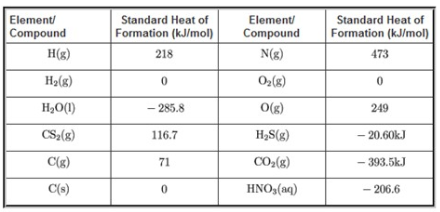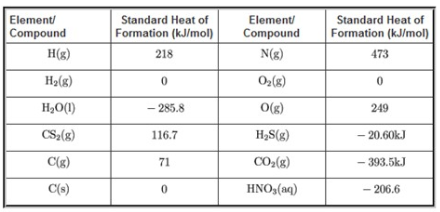# Problem: What is ΔHrxn° for the following chemical reaction? CS2(g) + 2H2O(l) → CO2(g) + 2H2S(g) You can use the following table of standard heats of formation (ΔH f°) to calculate the enthalpy of the given reaction.Express the standard enthalpy of reaction to three significant figures and include the appropriate units.

###### FREE Expert Solution

Recall that the equation for ΔH°rxn is:

$\overline{){\mathbf{∆}}{\mathbit{H}}{{\mathbf{°}}}_{{\mathbf{rxn}}}{\mathbf{=}}{\mathbf{\left(}}{\mathbit{p}}{\mathbit{r}}{\mathbit{o}}{\mathbit{d}}{\mathbit{u}}{\mathbit{c}}{\mathbit{t}}{\mathbit{s}}{\mathbf{\right)}}{\mathbf{-}}{\mathbf{\left(}}{\mathbit{r}}{\mathbit{e}}{\mathbit{a}}{\mathbit{c}}{\mathbit{t}}{\mathbit{a}}{\mathbit{n}}{\mathbit{t}}{\mathbit{s}}{\mathbf{\right)}}}$

For this problem, we need to solve for ΔH°rxn for the reaction:

CS2(g) + 2H2O(l) → CO2(g) + 2H2S(g)

Upon checking, the equation is balanced

We will use the following table for the standard heats of formation, ΔH°f :97% (494 ratings)###### Problem Details

What is ΔHrxn° for the following chemical reaction?

CS2(g) + 2H2O(l) → CO2(g) + 2H2S(g)

You can use the following table of standard heats of formation (ΔH f°) to calculate the enthalpy of the given reaction.Express the standard enthalpy of reaction to three significant figures and include the appropriate units.